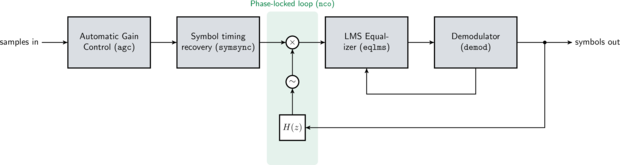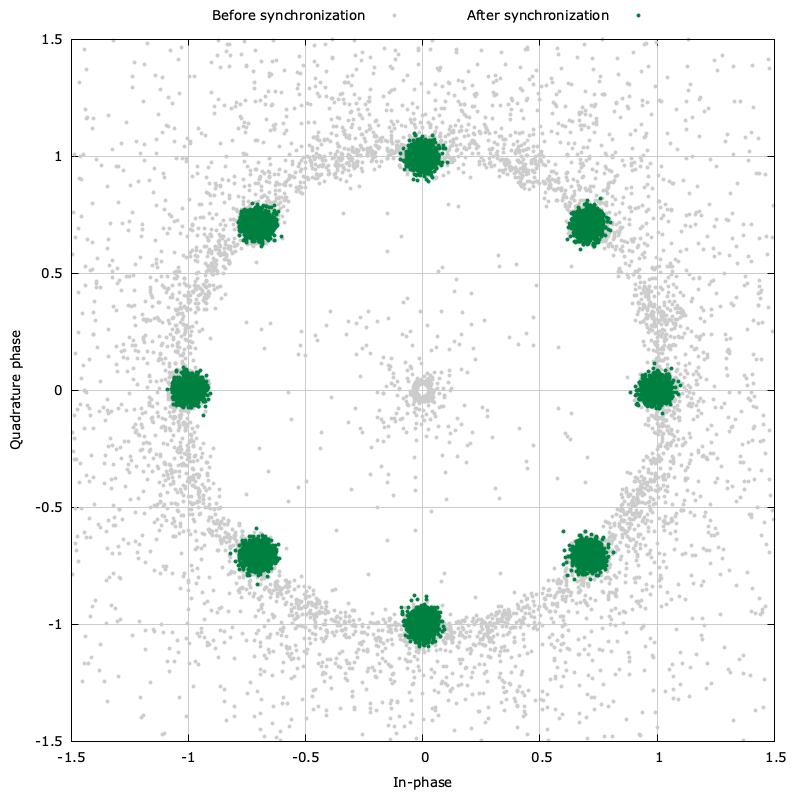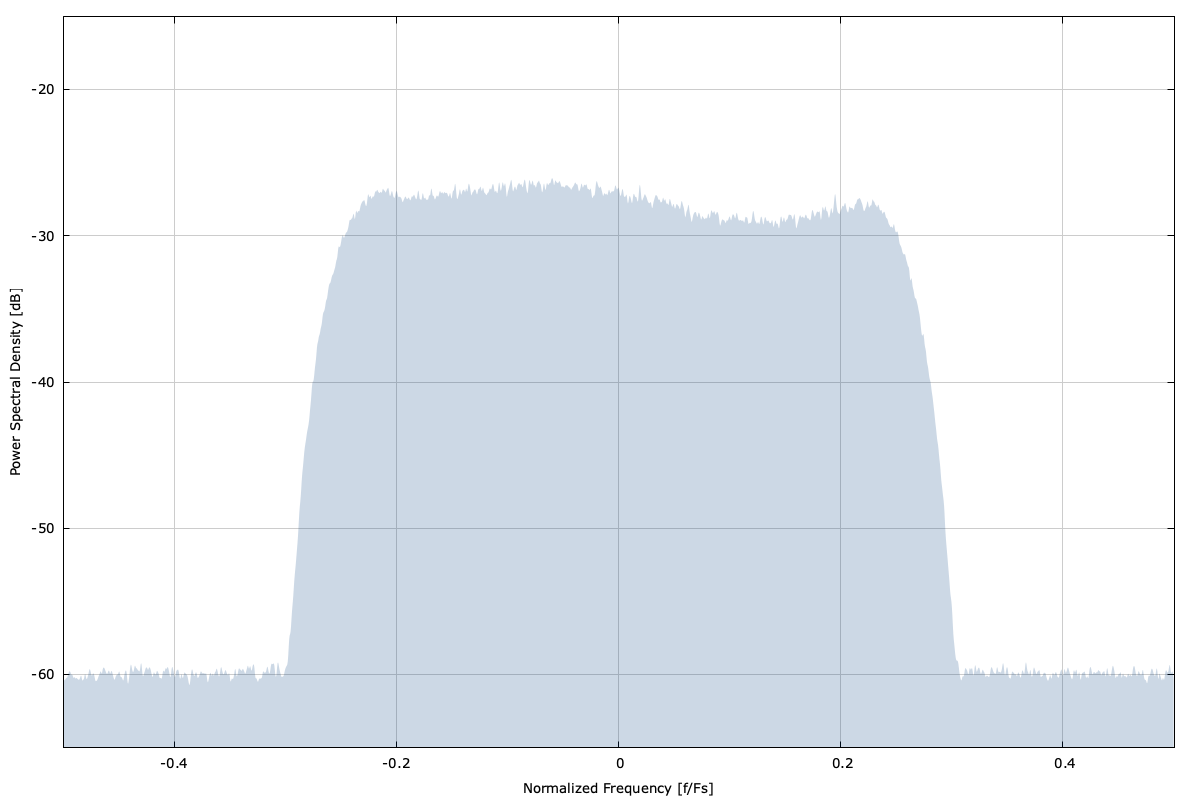# Symbol Tracker (symtrack)Figure [fig-symtrack-diagram]. symtrack (symbol synchronizer) functional block diagram

The symtrack object is a multi-rate symbol timing synchronizer as well as a carrier frequency/phase recovery and equalizer, useful for tracking a continuous stream of modulated symbols. The symtrack object recovers and corrects for the following unknowns:

• signal level
• sample timing (and slight symbol rate offsets)
• carrier phase and frequency
• channel multi-path

and provides a stream of output symbols ready for demodulation. It is effectively the same as the symsync object, but includes an internal control mechanism for also tracking carrier frequency and phase offsets simultaneously with an additional equalizing stage to remove inter-symbol interference from channel multi-path effects.

Shown below is an example of the symtrack objectFigure [fig-symtrack-time]. symtrack output constellationFigure [fig-symtrack-freq]. Power spectral density

Figure [fig-symtrack]. symtrack (symbol synchronizer) demonstration for a 8-PSK signal with a square-root Nyquist pulse with $$k=2$$ samples/symbol, a delay of $$m=7$$ symbols, and an excess bandwidth factor $$\beta=0.2$$

Here is a basic example program demonstrating how to use the object:

#include <liquid/liquid.h>

int main() {
// options
int          ftype = LIQUID_FIRFILT_RRC; // filter type
unsigned int k     = 2;                  // samples/symbol
unsigned int m     = 3;                  // filter delay (symbols)
float        beta  = 0.3f;               // filter excess bandwidth factor
int          ms    = LIQUID_MODEM_QPSK;  // modulation scheme (can be unknown)

// create symbol tracking synchronizer
symtrack_cccf symtrack = symtrack_cccf_create(ftype,k,m,beta,ms);

unsigned int  buf_len = 256;    // number of input samples
float complex buf_in [buf_len]; // complex input
float complex buf_out[buf_len]; // output buffer
unsigned int  num_written;      // number of values written to buffer

// ... initialize input buffer with data ...

// execute symbol tracker, storing result in output buffer
symtrack_cccf_execute_block(symtrack,
buf_in,  buf_len,
buf_out, &num_written);

printf("symtrack wrote %u symbols from %u input samples\n",
num_written, buf_len);

// ... repeat as necessary ...

// clean up allocated objects
symtrack_cccf_destroy(symtrack);
}


For a more detailed example, refer to examples/symtrack_cccf_example.c located under the main liquid project source directory.